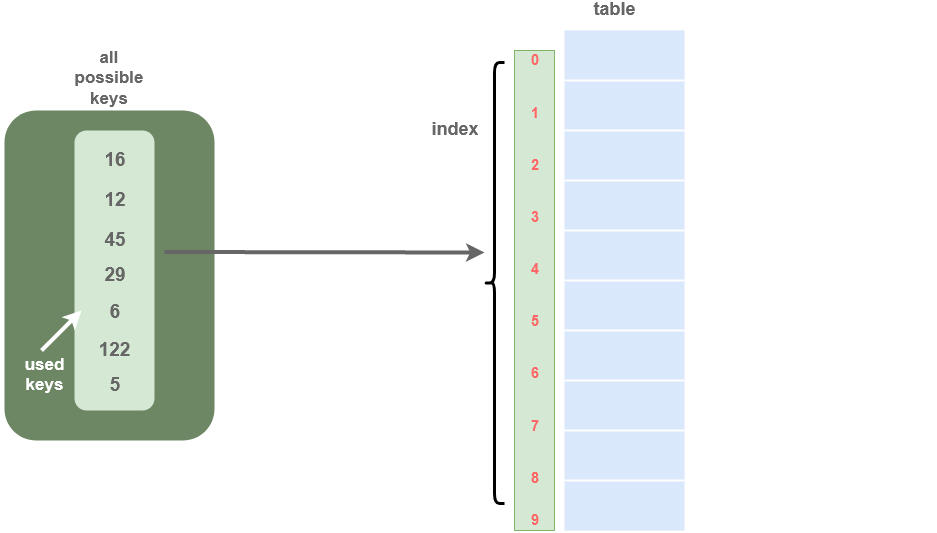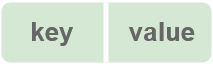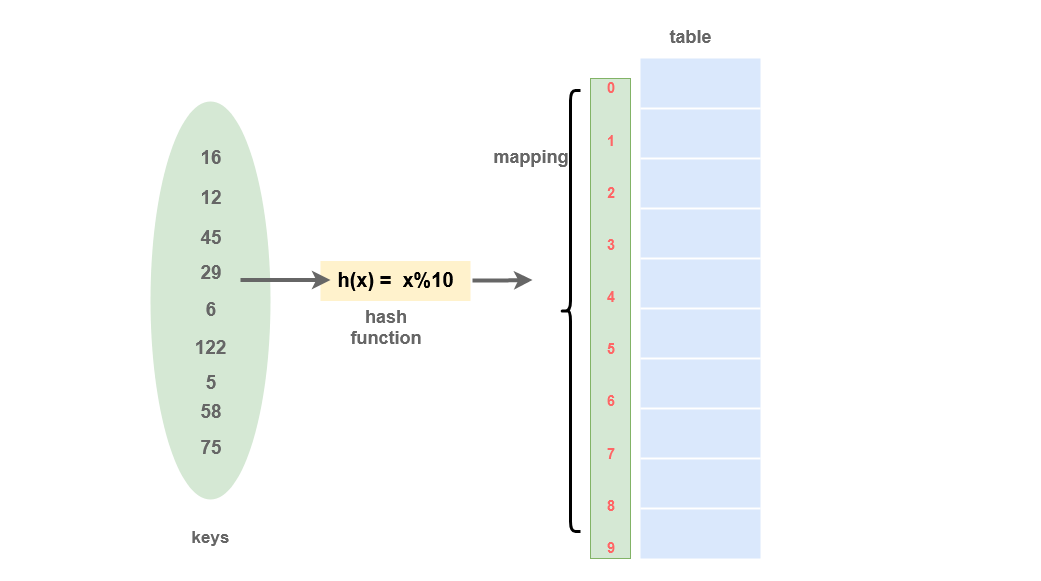# Hash Tables

Hash tables is a data structure which is used to map a set of keys to its corresponding values. They are a very common data structure and multiple problems use hash table directly/indirectly to solve multiple problems.

There are primarily two types of hash tables:

## Direct Address Table

Direct Address Table is a data structure in which data is mapped to keys using arrays. The table can be a big array if there are a small number of possible unique keys.
Let the universe of N possible keys be U = {0, 1, .., N-1}, then, the Direct-Address Table T[0,.., N-1] is an array where each slot (array element) corresponds to a unique key. Sometimes, the key itself is the data.

We use the direct address table when the program is not affected by the space used by the table. It is assumed that there are a small number of keys which are unique small integers.• Searching: In Direct address tables, arrays are used, so, the key values (which are also the index of the array) can be easily used to search the records in `O(1)` time.
• Insertion: Elements can easily be inserted in an array in `O(1)` time. The same thing follows in a direct address table also.
• Deletion: Deletion of an element takes `O(1)` time in an array. Similarly, to delete an element in a direct address table, we need `O(1)` time.

### Drawback of Direct Address Table

If there are N possibilities of the keys in the direct access table, then memory is created for all the N possible keys.
It may be possible that, in actuality, there is a deficient number of keys that are needed to be stored in the created table.

For example, If a Direct Address Table is created for a universe of 100 keys.
Suppose it is required to store only ten keys in the table. The rest of the 90 places will be left vacant in this table. So, this is the major drawback of using the Direct Address Table.

## Hashing

Hashing is a procedure in which a given set of keys are converted into some another value using a mathematical algorithm with the help of a hash function. The result of a hash function is known as a hash value or simply a hash. For each data, a key is assigned and based on which operations are performed on the data.

Hashing is used to store data into a data structure in a way such that the operations like insertion, deletion, and searching are performed in O(1) time.

## Hash Table

Hash Table is a data structure used to store data based on a key generated by a Hash function that takes the data as input and produces a key.

The keys are processed to produce a new index that maps to the required element.In a Hash table, data is represented in a key-value pair with the help of keys. Each data is associated with a particular key. The key is an integer that points to the data.• Searching: The hash function can be used to search the index of particular data. The hash function returns the index where the data is being stored for any required data. So, the searching operation can be performed in `O(1)` time.
• Insertion: A hash function will generate a key in O(1) time. So, the overall time complexity of the insertion of data in the hash table is `O(1)`.
• Deletion: For the deletion of an element from the hash table, the hash function can be used to find the position of the element in the hash table and go directly to that place and directly delete that item. So, the overall deletion operation can be performed in `O(1)`.

### Drawbacks of Hash Table

One of the drawbacks of the hash function is that if it produces the same index for multiple keys, then conflict arises. This situation is known as a collision.
Collisions can be removed by having uniformly distributed keys, and this can be achieved by using a proper hash function.

For example, If `k` is a key and `N` is the size of the hash table, the hash function `h(k)` :

`h(k) = k mod N` is a good hash function.

However, it is impossible to produce all unique keys. This can be used to reduce the number of collisions, but it is not sufficient it preventing them.

## Collision resolution techniques

Since multiple keys can be mapped to same hash value, there are high chances of collision in a hash table. There are two common collision resolution techniques which are used in hash tables:

Scroll to Top
[gravityforms id="5" description="false" titla="false" ajax="true"]